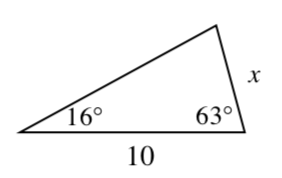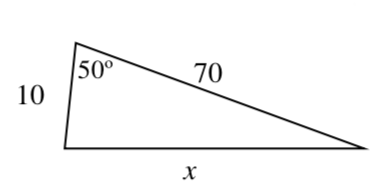### Home > INT3 > Chapter 12 > Lesson 12.1.4 > Problem12-69

12-69.

While working on homework, Zachary is calculating the value of $x$ in each of the diagrams below. His first step for each problem is shown under the diagram. If his first step is correct, continue solving the problem to determine the solution. If his first step is incorrect, explain his mistake and solve the problem correctly.

1.$\sin\left(16^{\circ}\right)=\frac{x}{10}$

This is not a right triangle so use the Law of Sines.

1.$x^2=10^2+70^2-2\left(10\right)\left(70\right)\cos\left(50^\circ\right)$

This method is correct $x ≈ 64$ units